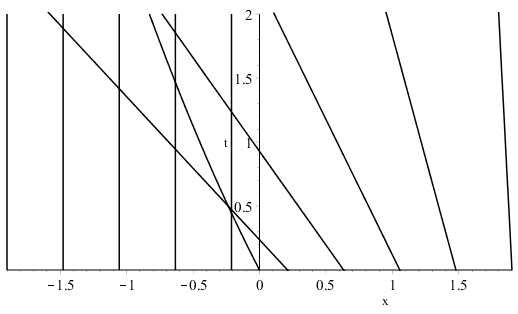# Finding the time for the first shock for a quasilinear first order PDE

• I
• BloonAinte

#### BloonAinte

TL;DR Summary
To find a shock wave, do we always solve the equation ##x_{\xi}=0##? The PDEs I consider are of the form ##u_t + g(u) u_x = f(u)##, with initial condition ##u(x,0) = h(x)##.
To find a shock wave, do we always solve the equation ##x_{\xi}=0##? The PDEs I consider are of the form ##u_t + g(u) u_x = f(u)##, with initial condition ##u(x,0) = h(x)##. I have been looking at the solutions for problems in my homework sheet but this method was used with no explanation.

Why does this method work to find the formation of the first shock?

Thank you very much!

A shock forms when neighbouring charcteristics first intersect. Characteristics corresponding to neighbouring values of $\xi$ will intersect when $$(x(\xi,t),t) = (x(\xi + \delta \xi, t), t)$$ which to first order in $\delta \xi$ requires $$\frac{\partial x}{\partial \xi} = 0.$$

•BloonAinte
Thank you! Does this method always determine the first shock?

I have looked up an example to illustrate my doubt:

https://math.stackexchange.com/ques...-curve-and-sketch-characteristics-in-xt-plane

That question considers some PDE and obtains characteristics as follows:In this question, the characteristics for ##x > 0.5## intersect those for ##x < -0.5##. These are not neighbouring characteristics, but they have intersections. However, ##t## is not minimal there, so it's not the position of the first shock. These also do not seem to solve ##x_{\xi} = 0##.

From this "investigation", I guess that this means that this method of setting ##x_{\xi}=0## will always give the position of the first shock, and this always occurs due to characteristics which are close together.

Does this sound accurate? Thank you!

This example is complicated by the fact that $x_\xi$ does not exist at $\xi = 0$. In this case, a characteristic in $\xi > 0$ intersects the characteristic $x = 0$ when $$t = \frac{2\xi}{2 - \xi}.$$ This first happens at $$\max \left\{ 0, \inf_{\xi \geq 0} \frac{2\xi}{2 - \xi} \right\} = 0.$$

•BloonAinte
Thank you so much for all your help! :) I understand this more now ^^

•jim mcnamara